## Solutions of Electricity Lakhmir Singh Manjit Kaur SAQ, LAQ, HOTS and MCQ Pg No. 43 Class 10 Physics36. The figure given below shows three resistors
Their combined resistance is:A. 7.5 Ω
B. 14 Ω
C. 10 Ω
D. 15 Ω

→ Here two resistor 6 ohm and 2 ohm are connected in parallelNow R ohm and 6 ohm are in series
So the combined resistance37. If two resistors of 35 Ω and 15 Ω are joined together in series and then placed in parallel with a 40 Ω resistor, the effective resistance of the combination is :
A. 0.1 Ω
B. 10 Ω
C. 20 Ω
D. 40 Ω

→ When the resistors are in series, then the combined resistance = 35 Ω+15 Ω = 50Ω
Now 50Ω and 40 Ω are connected in parallel, so the combined resistance38. The diagram below shows part of a circuit:If this arrangement of three resistors was to be placed by a single resistor, its resistance should be :
A. 9 Ω
B. 4 Ω
C. 6 Ω
D. 18 Ω

→ The two 6 Ω resistors are in parallel. Hence, the Equivalent Resistor =Now, 3 Ω and 6 Ω resistors are in series. Hence the Total Equivalent Resistance = 3 + 6 = 9 Ω

39. In the circuit shown below :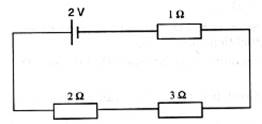The potential difference across the 3 Ω resistor is:
A. 5V
B. 4V
C. 1V
D. 2V

→ Here all the resistance are connected in series. Therefore net resistance = 2Ω+3Ω+1Ω =6Ω
So current in the circuit =Potential across 3Ω resistor =40. A battery and three lamps are connected as shown:Which of the following statements about the currents at X, Y and Z is correct?
A. The current at Z is greater than that at Y.
B. The current at Y is greater than that at Z.
C. The current at X equal the current at Y.
D. The current at X equals the current at Z.

→ The current at Y is greater than that at Z due to the arrangement of the circuit.

41. V1, V2 and V3 are the p.d. across the 1 Ω, 2 Ω and 3 Ω resistors in the following diagram, and the current is 5A.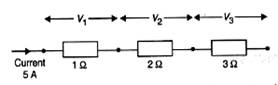Which one of the columns (a) to (d) shows the correct values of V1, V2 and V3 measured in volts?
A. V1- 1.0, V2- 2.0, V3- 3.0
B. V1- 5.0, V2-10.0, V-15.0
C. V1- 5.0, V2-2.5, V3- 1.6
D. V1- 4.0, V2-3.0, V3- 2.0

→ As V = IR

42. A wire of resistance R1 is cut into five equal pieces. These five pieces of wire are then connected in parallel. If the resultant resistance of this combination be R2, then the ratio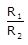is:
A. 20:21
B. 25:1
C. 5:19
D. 25:7

→ When the wire is cut into 5 pieces, resistance of each part is R/5
So, equivalent resistance of the 5 pieces when connected in parallel isR1 = R/25
So required ratio R: R1=25:1

Questions Based on High Order Thinking Skills (HOTS)-Pg-43

43. Show with the help of diagrams, how you would connect three resistors each of resistance 6 Ω so that the combination has resistance of (i) 9 Ω (ii) 4 Ω

→ (i)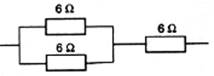To obtain 9Ω resistance two resistance are connected in parallel,
Let the resultant resistance for parallel circuit=R
1/R=1/6+1/6
1/R=2/6
R=3
Effective resistance=6+3 = 9 ohms
(ii) To obtain 4Ω resistance all resistance are connected in parallel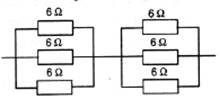So the effective resistance will be R for each circuit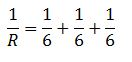Or R= 2 Ω
Total resistance = 2 Ω+2 Ω=4Ω

44. To resistances when connected in parallel give resultant value of 2 ohm; when connected in series the value becomes 9 ohm. Calculate the value of each resistance.

R1+R2=9
R2= 9-R1R21-9R1+18=0
(R1-3)(R1-6)=0
R1=3,6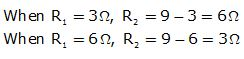45. A resistor of 8 ohm. Calculated in parallel with another resistor X. The resultant resistance of the combination is 4.8 ohms. What is the value of the resistor X?

→ Parallel combinations of the resistors will be written as
1/x + 1/8 = 1/4.8
On solving the above relation,
x = 12 ohms

46. You are given three resistances of 1, 2 and 3 ohms. Show by diagrams, how with the help of these resistances you can get :
(i) 6 Ω (ii) C (iii) 1.5 Ω

→ (i) 6 ohm can be obtained by connecting the 1,2 and 3 ohm in series.(ii) 6/11 ohm can be obtained by connecting the 1,2 and 3 ohm in parallel connection.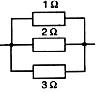(iii) 1.5 ohm can be obtained by connecting 1 and 2 ohm in series and then by connecting 3 ohm to the resultant resistance i.e 3 ohm in parallel connection.47 How will you connect three resistors of 2Ω, 3Ω and 5Ω respectively so as to obtain a resultant resistance of 2.5 Ω? Draw the diagram to show the arrangement.

→ Resistor 2 and 3 ohm are connected in series, 5 ohm resistance is connected parallel to 2 and 3 ohm.
Thus the resultant resistance will be 2.5 ohm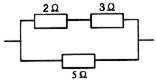48. How will you connect three resistors of resistance 2Ω, 3 Ω and 6 Ω to obtain a total resistance of: (a) 4Ω, and (b) 1 Ω?

→ (a) For total Resistance to be 4
we will connect 2 ohm resistance in series with parallel combination of 3 and 6 ohm resistance. When 3 and 6 ohm are in parallel combination then 1/R=1/3+1/6
or R=2
Now this R connected in series with 2 ohm resistance will give equivalent resistance = 2+ 2 = 4 ohm.
For total Resistance to be 1 ohm
All the given resistance should be connected in parallel combination 1/R’= 1/2+1/3+1/6
Or R'=1 ohm

49. What is (a) highest, and (b) lowest, resistance which can be obtained by combining four resistors having the following resistances?
4Ω, 8Ω, 12Ω, 24Ω

→ (a) To obtain the highest resistance we must connect the given resistances in series.
Highest resistance R = 4 + 8 + 12 + 24 = 48 ohms
(b) To obtain the lowest resistance we must connect the given resistances in parallel.
1/R=1/4+1/8+1/12+1/24
On solving we get, R=2 ohms.

50. What is the resistance between A and B in the figure given below?→ According to the figure, 20 ohm, 10 ohm and 20 ohm are connected in series so the resultant resistance in the extreme side = 20+10+20 = 50 ohms
Now this 50 ohm is in parallel with 30 ohm, so the resultant resistance become
On solving we get R = 18.75 ohm
Now 18.75 ohm, 10 ohm and 10 ohm are connected in series. So the resultant resistance becomeR= 18.75+10+10 = 38.75 ohm.

51. You are given one hundred 1 Ω resistors. What is the smallest and largest resistance you can make in the circuit using these?

→ The greatest possible resistance is the series combination of the resistors.
In series combination of hundred 1 ohm resistors equivalent resistance will be 100 ohm
The least possible resistance is the parallel combination of the resistors.
When resistances are connected in parallel then
1/R =100
R= 1/100 =0.01 ohm

52. You are supplied with a number of 100 Ω resistors. How could you combine some of these resistors to make a 250 Ω resistor?

→ To make equivalent resistance of 250 ohms one may connect:
1) 2 resistors in series Thus, the resultant would be:
100+100= 200 ohms
2) 2 resistors in parallel Thus, the resultant of 2 parallel combination would be: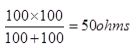3) Now connect the combination of the two (series and parallel) in series: Thus, we may obtain a net resistance of: 200+50 = 250 ohms.

53. The resistors R1, R2, R3 and R4 in the figure given below are all equal in value.What would you expect the voltmeters A, B and C to read assuming that the connecting wires in the circuit have negligible resistance?54. Four resistances of 16 ohms each are connected in parallel. Four such combinations are connected in series. What is the total resistance?

→ When four 16 ohm resistances is connected in parallel combination then the equivalent resistance will be
1/R = 1/16 + 1/16 + 1/16 + 1/16 = 4/16
R = 4 ohm
When four such combinations are connected in series, than the total resistance = 4+4+4+4 = 16 ohm.

55. If the lamps are both the same in the figure given below and if A1 reads 0.50 A, what do A2, A3, A4 and A5 read?

→ It is given that the two lamps are identical. So, they will have equal resistance.
The ammeter A1 reads 0.5 A current. This current will now get distributed equally in the two branches as resistances are equal.
Hence, the ammeters A2 and A4 will read 0.25 A each.
Now, A3 and A5 are connected in series with A2 and A4, so they will also read 0.25 A.

1. Are the lights in your house wired in series?

→ No, When appliances are connected in a parallel arrangement, each of them can be put on and off independently. This is a feature that is essential in a house's wiring.

2. What happens to the other bulbs in a series circuit if one bulb blows off?

→ When bulbs are connected in a series arrangement, if one of them can be put off all bulbs are switched off.

3. What happens to the other bulbs in a parallel circuit if one bulb blows off?

→ When bulbs are connected in a parallel arrangement, each of them can be put on and off independently.

4. Which type of circuit, series of parallel, is preferred while connecting a large number of bulbs:
(a) for decorating a hotel building from outside?
(b) for lighting inside the rooms of the hotel?

→ (a) For decorating a hotel building from outside we use series connection.
(b) For lighting inside the rooms of the hotel we use parallel connection.

5. Draw a circuit diagram to show two 4 V electric lamps can be lit brightly from two 2 V cells.6. Why is series arrangement not used for connecting domestic electrical appliances in a circuit?

→ In series arrangement if one electrical appliance stops working due to some defect, then all other appliance also stop working as the whole circuit is broken.So we should not used series arrangement for connecting domestic electrical appliances in a circuit.

7. Give three reasons why different electrical appliances in a domestic circuit are connected in parallel.

→ Due to the following advantages different electrical appliances in a domestic circuit are connected in parallel:
(i) Each electrical appliance gets the same voltage as that of the power supply line.
(ii) When appliances are connected in a parallel arrangement, each of them can be put on and off independently.
(iii) If one electrical appliance stops working due to some defect, then all other appliances keep working properly.

8. Ten bulbs are connected in a series circuit to a power supply line. Ten identical bulbs are connected in a parallel circuit to an identical power supply line.
(a) Which circuit would have the highest voltage across each bulb?
(b) In which circuit would the bulbs be brighter?
(c) In which circuit, if one bulb blows out, all others will stop glowing?
(d) Which circuit would have less current in it?

→ (a) Parallel circuit would have the highest voltage across each bulb.
(b) In Parallel circuit the bulbs will be brighter.
(c) In series circuit, if one bulb blows out, all others will stop glowing
(d) Series circuit would have less current in it.

9. Consider the circuits given below:(a) In which circuit are the lamps dimmest?
(b) In which circuit or circuits are the maps or equal brightness to the lamps in circuit (i)?
(c) Which circuit gives out the maximum light?

→ (a) In circuit (ii) the lamps are dimmest as it is connected in series arrangement.
(b) In circuit (iii) the lamps are equal brightness in circuit (i) as both are connected in parallel.
(c) Circuit (ii) give the maximum light.

10. If you were going to connect two light bulbs to one battery, would you use a series or a parallel arrangement? Why? Which arrangement takes more current from the battery?

→ If we were going to connect two light bulbs to one battery, we would use a parallel arrangement, so the current will flow through them equally. Series arrangement take more current from battery.

11A. Which is the better way to connect light and other electric appliances in domestic wiring: series circuits or parallel circuits? why?

→ When appliances are connected in a parallel arrangement, each of them can be put on and off independently. This is a feature that is essential in a house's wiring.

11B. Christmas tree lamps are usually wired in series. What happens if one lamp breaks?

→ In series circuit, if one bulb blows out, all others will stop glowing.

11C. An electrician has wired a house in such a way that if a lamp gets fused in one room of the house, all the lamps in other rooms of the house stop working. What is the defect in the wiring?

→ The circuit arrangement is in series, so if one bulb blows out, all others will stop glowing.

11D. Draw a circuit diagram showing two electric lamps connected in parallel together with a cell and a switch that works both lamps. Mark an (A) on your diagram to show where an ammeter should be placed to measure the current.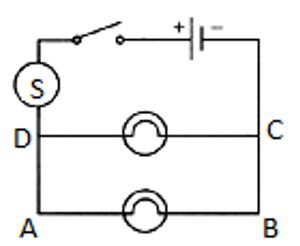Multiple Choice Questions (MCQs)-Pg-48

12. The lamps in a household circuit are connected in parallel because:
A. this way they require less current
B. if one lamp fails the others remain lit
C. this way they require less power
D. if one lamp fails the others also fail

→ The lamps in a household circuit are connected in parallel because in a parallel arrangement, each of them can be put on and off independently.

13. Using the circuit given below, state which of the following statement is correct?A. When S1and S2 are closed, lamps A and B are lit.
B. With S1open and S2 closed, A is lit and B is not lit.
C. With S2 open and S1 closed A and B are lit.
D. With S1 closed and S2 open, lamp A remains lit even if lamp B gets fused.

→ When S2 open and S1 closed A and B are lit.

Questions Based on High Order Thinking Skills (HOTS)-Pg-48

14A. Draw a circuit diagram showing two lamps, one cell and a switch connected in series.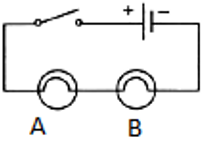14B. How can you change the brightness of the lamps?

→ When lamps are connected in parallel the brightness of the lamps increases.

15. Consider the circuit given below where A, B and C are three identical light bulbs of constant resistance.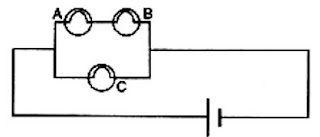(a) List the bulbs in order of increasing brightness.
(b) If C burns out, what will be the brightness of A now compared with before?
(c) If B burns out instead, what will be the brightness of A and C compared with before?

→ (a) Here A and B are connected in series so the brightness of the A and B will same while C are connected in parallel so the brightness of the bulb C is maximum.
(b) Their will be no effect on A and B.
(c) A and B are connected in series so if B burns than A also not glow but their will be no effect on C, as C is connected in parallel.

16. How do you think the brightness of two lamps arranged in parallel compares with the brightness of two lamps arranged in series (both arrangements having one cell)?

→ The brightness of two lamps which are arranged in parallel is maximum than the the brightness of two lamps arranged in series.

17. If current flows through two lamps arranged:
(a) in series,
(b) in parallel,
and the filament of one lamps breaks, what happens to the other lamp? Explain your answer

→ (a) In series circuit, if one bulb blows out, all others will stop glowing.
(b) When lamps are connected in a parallel arrangement, each of them is independently.

18. The figure below shows a variable resistor in a dimmer switch.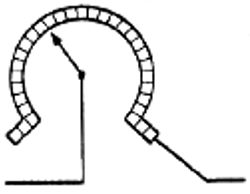How would you turn the switch to make the lights:
(a) brighter, and
(b) dimmer?

→ (a) To make the light brighter we turn the switch towards right side.
(b) To make the light dimmer we turn the switch towards left side.

1. State two factors on which the electrical energy consumed by an electrical appliance depends.

→ Two factors on which the electrical energy consumed by an electrical appliance depends on.
1) Power rating of the appliance.
2) Time for which the appliance is used.

2. Which one has a higher electrical resistance: a 100-watt bulb or a 60 watt bulb?

→ As we know thatP is inversely proportional to resistance, so 60 watt has a higher electrical resistance.

3. Name the commercial unit of electric energy.

→ The commercial unit of electric energy is Kilowatt-hour.

4. An electric bulb is rated at 220 V, 100 W. What is its resistance?

→ Here, V = 220 V, P = 100W
R = ?
We know thatThus
R = V2/P = (220)2/100 = 484 ohm.

5. What is the SI unit of (i) electric energy, and (ii) electric power?

→ (i) The SI unit of electric energy is joule.
(ii) The SI unit electric power is watt.

6. Name the quantity whose unit is (i) kilowatt, and (ii) kilowatt-hour.

→ (i) Kilowatt is the S.I unit of Electric power.
(ii) kilowatt-hour is the S.I unit of Electric energy.

7. Which quantity has the unit of watt?

→ Watt is the SI unit of Electric power.

8. What is the meaning of the symbol kWh? Which quantity does it represent?

→ kWh is the commercial unit of electrical energy. It full form is kilowatt-hour.

9. If the potential difference between the end of a wire of fixed resistance is doubled, by how much does the electric power increase?

→ As we know thati.e. as power is directly proportional to the square of potential difference. So, the electric power becomes four times its previous value.10. An electric lamp is labeled 12 V, 36 W. This indicates that it should be used with a 12 V supply. What other information does the label provide?

→ The electric lamp consumes energy at the rate of 36 J/s.

11. What current will be taken by a 920 W appliance if the supply voltage is 230 V.

→ Here P = 920W, V = 230V, I = ?
We know that
P = V x I
920 = 230 x I
I = 920/230 = 4amp

12. Define watt. Write down an equation linking watts, volts and amperes.

→ One watt is defined as the amount of electrical energy consumed by electrical appliances at the rate of 1 joule per second.
1 watt = 1 volt x 1 ampere

13. Define watt-hour. How many joules are equal to 1 watt-hour?

→ The amount of electrical energy consumed by an electrical appliance of 1 watt power for 1 hour is called watt-hour.
1 watt hour is equal to 3600 joules

14. How much energy is consumed when a current of 5 amperes flows through the filament (or element) of a heater having resistance of 100 ohms for two hours? Express it in joules.

→ Here, current (I) = 5amp, Resistance(R) = 100 ohms and time = 2h
As we know that
Electric energy consumed = P x t = I2Rt
= 52 x 100 x 2
= 5000 Wh
= 5 kwh
We know that
1kwh = 3.6 x 106 J
Therefore, 5kwh = 5 x 3.6 x 106 J = 18 x 106 J

15. An electric bulb is connected to a 220 V power supply line. If the bulb draws a current of 0.5 A, calculate the power of the bulb.

→ Here, Potential difference (V) = 220V, Current (I) = 0.5amp, Power = ?
We know that
P = VI = 220 X 0.5
P = 110 watt.

16. In which of the following cases ore electrical energy is consumed per hour?
(i) A current of 1 ampere passed through a resistance of 300 ohms.
(ii) A current of 2 amperes passed through a resistance of 100 ohms.

→ (i) Here current (I) = 1 A, Resistance (R) = 300 ohm, time(t) = 1h
We know that
P = I2R = 12 x 300 = 300 W
E = P x t = 300 x 1 = 300 Wh
(ii) Here Current (I) = 2 A Resistance(R) = 100 ohm, time (t) = 1h
We know that
P = I2R = 22 x 100 = 400 W
E = P x t = 400 x 1 = 400 Wh
Hence, in case (ii), the electrical energy consumed per hour is more.

17. An electric kettle rated at 220 V, 2.2 kW, works for 3 hours. Find the energy consumed and the current drawn.

→ Here potential difference(V) = 220V, Power (P) = 2.2kW = 2200W, time(t) = 3h
We know that
Electrical energy consumed = P × t = 2.2 ×3 = 6.6 kWh
We have, P = V x I
I = P/V
I = 2200/220
= 10amp

18. In a house two 60 W electric bulbs are lighted for 4 hours, and three 100 W bulbs for 5 hours every day. Calculate the electric energy consumed in 30 days.

→ When two electric bulb of 60W are lighted for 4 hours then
Here, p1 = 60w
Number, n1 = 2
Time for daily use, t1 = 4h
Total electrical consumed everyday, E1 = n1×p1×t1
= 2×60×4 = 480
Energy consumed in 30 days = 30×0.48 = 14.4Kwh (As 1kW=1000W)
When three electric bulb of 100W are lighted for 5 hours then
Here, p2 = 100w
Number, n2 = 3
Time for daily use, t2 = 5h
Total electrical consumed every day, E1 = n2×p2×t2
= 3×100×5 = 1500
Energy consumed in 30 days = 30× 1.5 = 45Kwh (As 1kW= 1000 W)
Total electrical consumed in 30 days = 14.4Kwh + 45Kwh = 59.4 kWh

19. A bulb is rated as 250 V; 0.4 A Find its:
(i) power, and (ii) resistance.

→ (i) We know that
V = 250V, I = 0.4amp
Power = VI = 250X0.4 = 100watt
(ii) We have,
V = 250V, I = 0.4amp
We know that
P = I2R
100 = (0.4)2XR
R = 625 ohm

20. For a heater rated at 4 kW and 220 V, calculate:
(a) the current,
(b) the resistance of the heater,
(c) the energy consumed in 2 hours, and
(d) the cost if 1 kWh is priced at ₹ 4.60

→ (a) Here, P = 4kw, V = 220v
I = ?
We know that
Power = VI = 220 × I
4000 = 220I
I = 4000/220= 18.18A
(b) Here, P = 4kw, V = 220v
R = ?
We know that,
P = I2R
P = (18.18)2×R
R = 4000/(18.18)2
R = 12.10 Ω
(c) Here, P = 4kw, time = 2hr
Energy consumed in two hour = P × t
= 4 × 2
= 8kw-hr
(d) If 1kwh = Rs 4.6
Here, Energy consumed in two hour = 8kwh
So, total cost = 8 x 4.6 = Rs 36.8

21. An electric motor take 5 amperes current from a 220 volts supply line. Calculate the power of the motor and electrical energy consumed by it in 2 hours.

→ Here current (I) = 5amp, Potential difference(V) = 220 volt, time = 2h,
We have to find
Power (P) = ?
Energy (E) = ?
We know that
P = V×I
= 220×5
= 1100 watt
= 1.1 kW
Energy consumed, E = P × t
= 1.1 × 2
= 2.2 kWh

22. Which users more energy: a 250 W TV set in 1 hour or a 1200 W toaster in 10 minutes?

→ TV set uses 0.25 kWh energy whereas toaster uses 1.20 kWh energy. So, toaster uses more energy.

23. Calculate the power used in the 2 Ω resistor in each of the following circuits:
(i) A 6V battery in series with 1 Ω and 2 Ω resistors.
(ii) A 4V battery in parallel with 12 Ω and 2Ω resistors.

→ (a) Here V = 6 volt, R1 = 1 ohm, R2 = 2 ohm
The connection is in series. So Equivalent resistance = R1+R2 = 1 + 2 = 3 ohm
We know that
Total current I = V/R = 6/3 = 2A
Current through R2 = I2 = I = 2A
Voltage across R2 = V2 = I2R2 = 2×2 = 4 ohm
Power used in R2 = I2V2 = 2×4 = 8W
(b) Here, V = 4 volt,R1 = 12 ohm, R2 = 2 ohm
We know that, as the connection is in parallel so voltage across R2 = V2 = V = 4V
Current across R2 = I2 = V2/R2 = 4/2 = 2A
As power = IV
Power used in R2 = I2V2 = 2×4 = 8W

24. Two lamps, one rated 40 W at 220 V and the other 60 W and 220 V, are connected in parallel to the electric supply at 220 V.
(a) Draw a circuit diagram to show the connections.
(b)Calculate the current drawn from the electric supply.
(b) Calculate the total energy consumed by the two lamps together when they operate for one hour.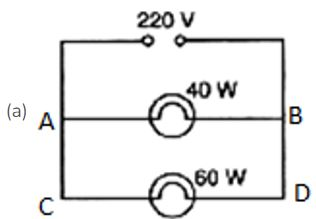(b) As the connection is in parallel.
So voltage across both the bulbs is same and is equal to 220V.
Using the Formula P=VI,We can get I as I=P/V Where:-
P= Power, I=Current, V=Voltage/Potential Difference
Current through AB i.e 40W lamp = I1 = P1/V = 40/220 A
Current through CD i.e. 60W lamp = I2 = P2/V = 60/220 A
Total current drawn from the electric supply = 40/220 + 60/220 = 0.45 A
(c)We know that Energy= Power x TimeTotal Power to two bulbs together= 40W+60W = 100W According to the question both of the bulbs are being operated together at 1 hour∴ Energy= 100W x 1hr Energy= 100Whr
We know 1Whr = 3600 Joules ∴100Whr = 3600 X 100 =360000Joules  =360kJoules.

25. An electric kettle connected to the 230 V mains supply draws a current of 10 A. Calculate:
(a) the power of the kettle.
(b) the energy transferred in 1 minute.

→ (a) Here, V = 230V, I = 10amp
We know that, P = VI
P = 230X10
P = 2300watt = 2300 J/s
(b) The energy transferred in 1 minute. = P x t = 2300 J/s x 60s = 138000 J

26. A 2 kW heater, a 200 W TV and three 100 W lamps are all switched on from 6 p.m. to 10 p.m. What is the total cost at Rs. 5.50 per kWh?

→ Here for heater we have, P = 2kW, t = 4h
So energy consumed by heater, E = P x t = 2x4 = 8kWh
Here for TV, we have, P = 200W = 0.2kW, time 10pm – 6pm = 4h
So energy consumed by TV, E = P x t = 0.2x4 = 0.8kWh
Here for TV, we have, P = 100W = 0.1kW, t = 4h, number of lamp(n) = 3
So energy consumed by three lamps, E = n x P x t = 3x0.1x4 = 1.2kWh
Total energy consumed by heater, TV and three lamps = 8+0.8+1.2 = 10kWh
Cost of 1kWh = Rs. 5.50
Cost of 10kWh = Rs. 5.50 x 10 = Rs. 55

27. What is the maximum power in kilowatts of the appliance that can be connected safely to a 13 A; 230 V mains socket?

→ Here I = 13amp, V = 230V
As we know that
Power = VI
= 230 x 13
= 2990W
P = 2.99kW

28. An electric fan runs from the 230 V mains. The current flowing through it is 0.4 A. At what rate is electrical energy transferred by the fan?

→ Here, V = 230V, I = 0.4amp
We know that power is the rate at which electric energy is transferred
P = V x I
= 230 x 0.4
= 92 W = 92 J/s

29A. What is meant by “electric power”? Write the formula for electric power in terms of potential difference and current.

→ The rate at which electrical energy is consumed, or the rate of doing electric work is known as electric power.
We know that
P = VI

29B. The diagram below shows a circuit containing a lamp L, a voltmeter and an ammeter. The voltmeter reading is 3 V and the ammeter reading is 0.5 A.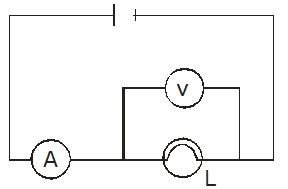(i) What is the resistance of the lamp?
(ii) What is the power of the lamp?

→ (i) resistance = V/I = 3/0.5 = 6 Ω
(ii) P = VI = 3 × 0.5 = 1.5 Watt

29C. Define kilowatt-hour. How many joules are the in one kilowatt-hour?

→ The amount of electrical energy consumed when an electrical appliance having a power rating of 1 kilowatt is used for 1 hour is called One kilowatt hour.
1kWh = 3.6 x 106J

29D. Calculate the cost of operating a heater of 500 W for 20 hours at the rate of ₹ 3.90 per unit.

→ Here, Power of heater (P) = 500W = 0.5kW, Time(t) = 20hr
We know that
Energy consumed = P x t = 0.5 X 20
= 10kwh
Total cost = 10Xcost per unit
Cost per unit = Rs. 3.9 per unit
Therefore, total cost = 10X 3.9 = Rs 39

Multiple Choice Questions (MCQs)-Pg-59

30. When an electric lamp is connected to 12 V battery, it draws a current of 0.5 A. The power of the lamp is :
A. 0.5 W
B. 6W
C. 12 W
D. 24W

→ We know that
P = VI
P = 12× 0.5 = 6W

31. The unit for expressing electric power is:
A. volt
B. joule
C. coulomb
D. watt

→ The unit for expressing electric power is watt

32. Which of the following is likely to be the correct wattage for an electric iron used in our homes?
A. 60 W
B. 250 W
C. 850 W
D. 2000 W

→ The correct wattage for an electric iron used in our homes is 850 W.

33. An electric heater is rated at 2kW. Electrical energy costs ₹ 4 per kWh. What is the cost of using the heater for 3 hours?
A. ₹ 12
B. ₹ 24
C. ₹ 36
D. ₹ 48

→ Energy consumed = P x t = 2 X 3 = 6kWh
Total cost = 6 x cost per unit
Cost per unit = Rs. 4 per kWh
Therefore, total cost = 6 x 4 = Rs 24

34. The SI unit of energy is:
A. joule
B. coulomb
C. watt
D. ohm-metre

→ The SI unit of energy is joule.

35. The commercial unit of energy is:
A. watt
B. watt-hour
C. kilowatt-hour
D. kilo-joule

→ The commercial unit of energy is kilowatt-hour.

36. How much energy does a 100 W electric bulb transfer in 1 minute?
A. 100 J
B. 600 J
C. 3600 J
D. 6000 J

→ Energy consumed = P x t = 100 ×60 = 6000 joule
As in 1 minute = 60 second.

37. An electric kettle for use on a 230 V supply is rated at 3000 W. For safe working, the cable connected to it should be able to carry at least:
A. 2 A
B. 5 A
C. 10 A
D. 15 A

→ As P = VI
I = P/V

38. How many joules of electrical energy are transferred per second by a 6V; 0.5 A lamp?
A. 30 J/s
B. 12 J/s
C. 0.83 J/s
D. 3 J/s

→ As P = VI

39. At a given time, a house is supplied with 100 A at 220 V. How many 75 W, 220 V light bulbs could be switched on in the house at the same time (if they are all connected in parallel)?
A. 93
B. 193
C. 293
D. 393

→ When they are connected in parallel 293 bulb will glow in the house at the same time.

40. If the potential difference between the ends of a fixed resistor is halved, the electric power will become:
A. double
B. half
C. four times
D. one-fourth

→ If the potential difference between the ends of a fixed resistor is halved, the electric power will become one-fourth.

Questions Based on High Order Thinking Skills (HOTS)-Pg-60

41. State whether an electric heater will consumer more electrical energy or less electrical energy per second when the length of its heating element is reduced. Given reasons for our answer.

→ An electric heater will consume more electrical energy as power is inversely proportional to the resistance.

42. The table below shows the current in three different electrical appliances when connected to the 240 V mains supply:(a) Which appliance has the greatest electrical resistance? How does the data show this?
(b) The lamp is connected to the mains supply by using a thin, twin-cored cable consisting of live and neutral wires. State two reasons why this cable should not be used for connecting the kettle to the mains supply.
(c) Calculate the power rating of the kettle when it is operated from the 240 V mains supply.
(d) A man takes the kettle abroad where the mains supply is 120 V. What is the current in the kettle when it is operated from the 120 V supply?

→ (a) Lamp has the greatest electrical resistance as resistance oppose the flow of current, and the lamp has least amount of current.
(b) According to the given data large amount of current is needed by kettle, so it must be connected to the earthing.
(c) As we know that
P = VI
V = 240V, I = 8.5A
P = 240X8.5 = 2040 W = 2.04 kW
(d) When kettle is connected to 240 V supply, then the P = 2040 W

43. A boy noted the readings on his home’s electricity meter on Sunday at 8 AM and again on Monday at 8 AM (see Figures below).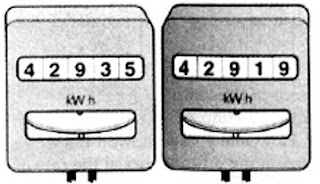(a) What was the meter reading on Sunday ?
(b) What was the meter reading on Monday?
(c) How many units of electricity have been used ?
(d) If the rate is Rs. 5 per unit, what is the cost of electricity used during this time?

→ (a) The meter reading on Sunday is 42919.
(b) The meter reading on Monday is 42935.
(c) Units of electricity used = 42935- 42919 = 16 units.
(d) The cost of 1 unit is Rs 5. So the cost of 16 units = 16×5 = 80 rupees

44. An electric bulb is rated as 10 W, 220V. How many of these bulbs can be connected in parallel across the two wires of 220 V supply line if the maximum current which can be drawn in 5A ?

→ Here it is given P = 10W, V = 220V, I = 5A
As we know that
P = VI
= 220X5
P = 1100W
Power of one bulb = 10W
So the total no. of bulbs that can be connected = 1100/10 = 110

45. Two exactly similar electric lamps are arranged (i) in parallel, and (ii) in series. If the parallel and series combination of lamps are connected to 220 V supply line one by one, what will be the ratio of electric power consumed by them?

→ As we know that
Electric power consumed = V2/R
Here V is given we have to find the value of R
So when the connection is in parallel
The equivalent resistance isElectric power consumed in parallel connectionWhen the connection is in Series
The equivalent resistance = R+R = 2R
Electric power consumed in series connection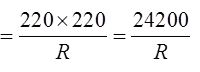The ratio of electric power consumed by them1. How does the heat H produced by a current passing through a fixed resistance wire depend on the magnitude of current I?

→ Heat produced, H = I2Rt
As heat produced is directly proportional to the square of current.

2. If the current passing through a conductor is doubled, what will be the change in heat produced?

→ Heat produced, H = I2Rt
So, If the current passing through a conductor is doubled then heat produced becomes four times.

3. Name two effects produced by electric current.

→ The two effects produced by electric current are:
(a) Heating effect of current
(b) Magnetic effect of current

4. Which effect of current is utilized in an electric light bulb?

→ Heating effect of current is utilized in an electric light bulb.

5. Which effect of current is utilized in the working of an electric fuse?

→ Heating effect of current is utilized in the working of an electric fuse.

6. Name two devices which work on the heating effect of electric current.

→ The two devices which work on the heating effect of electric current are Electric heater and electric fuse.

7. Name two gases which are filled in filament type electric light bulbs.

→ Argon and nitrogen are filled in filament type electric light bulbs.

8. Explain why, filament type electric bulbs are not power efficient.

→ Due to the heating effect of current lots of electric power is consumed by the filament of a bulb.

9. Why does the connecting cord of an electric heater not glow hot while the heating element does ?

→ Due to the low resistance of copper the connecting cord of the heater does not glow because negligible heat is produced in it by passing current but the heating element made of nichrome glows because it becomes red-hot due to the large amount of heat produced on passing current (because of its high resistance).

10A. Write down the formula for the heat produced when a current I is passed through a resistor R for time t.

→ The heat produced when a current I is passed through a resistor R for time t will be
Heat produced, H = I2Rt

10B. An electric iron of resistance 20 ohms draws a current of 5 amperes. Calculate the heat produced in 30 seconds.

→ Here,
R = 20ohm, I = 5amp, t = 30s
We know that H = I2Rt
H = 52X20X30
H = 15000 J

11. State three factors on which the heat produced by an electric current depends. How does it depend on these factors?

→ Heat produced by an electric current is given as H = I2Rt, that means
(i) Heat produced is directly proportional to square of current.
(ii) Heat produced is directly proportional to resistance.
(iii) Heat produced is directly proportional to the time for which current flows.

12A. State and explain Joule's law of heating.

→ Joule's law of heating states that when a current of I amperes flows in a wire of resistance R ohms for time t seconds then the heat produced in joules and is given by H = I2Rt
The law implies that heat produced in a resistor is (i) directly proportional to the square of current for a given resistance, (ii) directly proportional to resistance to resistance for a given current and (iii) directly proportional to the time for which current flows through the resistors.

12B. A resistance of 40 ohms and one of 60 ohms are arranged in series across 220 volt supply. Find the heat in joules produced by this combination of resistances in half a minute.

→ Here it is given that R1 = 40 ohms, R2 = 60 ohms, V = 220V, t = 30 sec
Here R1 and R2 are arranged in series connection.
So the equivalent resistance = R = 40+60 = 100 ohms
According to Ohm's law, we know that
V = IR
I = V/R
I = 220/100 = 2.2amp
As we know that heat produced in joules is given by H = I2Rt
Substituting the values of I, R and t in eq. H = I2Rt
H = (2.2)2 X 100 X 30
H = 14520 J

13. Why is an electric light bulb not filled with air? Explain why argon or nitrogen is filled in an electric bulb.

→ As the filament of the bulb is made from tungsten, so when we fill air inside the electric bulb this tungsten filament will burn quickly. So we fill non-reactive gas like argon or nitrogen in the electric bulb to prolong the life of filament.

14. Explain why, tungsten is used for making the filaments of electric bulbs.

→ Due to its very high melting point of tungsten it is used for making the filaments of electric bulbs.

15. Explain why, the current that makes the heater element very hot, only slightly warms the connecting wires leading to the heater.

→ Due to the low resistance of the connecting wires of the heater negligible heat is produced in them by passing current so it is slightly warm.

16. When a current of 4.0 A passes through a certain resistor for 10 minutes, 2.88x104 J of heat are produced. Calculate:
(a) the power of the resistor.
(b) the voltage across the resistor.

→ (a) Here it is given,
I = 4 amp,
t = 10 min = 10 x 60 = 600sec, H = 2.88x104J
We Know that according to Joule's law of heating
H = I2Rt
28800 = 42xRx600
R = 3ohms
We know that
P = I2xR
= 42x3
P = 48W
(b) Here we have to find the potential difference, V = ?
We know that
V = IR
V = 4x3
V = 12V

17. A heating coil has a resistance of 200 Ω. At what rate will heat be produced in it when a current of 2.5 A flows through it ?

→ Here Resistance of the coil(R) = 200 ohms, Current (I) = 2.5 amp, time(t) = 1 sec
We know that according to Joule's law of heating
H = I2Rt
H = (2.5)2×200×1
H = 1250 J/s

18. An electric heater of resistance 8 Ω takes a current of 15 A from the mains supply line. Calculate the rate at which heat is developed in the heater.

→ Here Resistance (R) = 8 ohms, Current(I) = 15 amp, time (t) = 1 sec
We know that according to Joule's law of heating
H = I2Rt
H = 152X8X1
H = 1800J/s

19. A resistance of 25 Ω is connected to a 12 V battery. Calculate the heat energy in joules generated per minute.

→ Here R = 25ohms, V = 12V, H = ?, t = 60sec
According to Ohm’s law
V = IR
12 = 25XI
I = 0.48amp
We know that according to Joule's law of heating
H = I2Rt
H = (0.48)2X25X60
H = 345.6J

20. 100 joules of heat is produced per second in a 4 ohm resistor. What is the potential difference across the resistor?

→ Here, it is given H = 100J, t = 1sec, R = 4ohms,
We know that according to Joule's law of heating
H = I2Rt
100 = (I)2×4×1
100/4 = I2
I = 5amp
Again we know that
V = IR
V = 5X4
= 20V

21A. Derive the expression for the heat produced due to a current 'I' flowing for a time interval 't' through a resistor 'R' having a potential difference 'V' across its ends. With which name is this relation known?

→ All materials offer resistance to the flow of current through them. So some external energy is required to make the current flow. This energy is provided by the battery. Some of this energy gets dissipated as heat energy, so the resistor becomes hot.
Work done in carrying a charge Q through a potential difference V is given as
Also, Q = I t
Using Ohm's law, V = I R
W = I2Rt
This work done in carrying the charge through the wire appears as the heat produced.
i.e. H = V I t =  I2Rt
this energy is dissipated as heat energy.
This law is called Joule law of heating effect.

21B. How much heat will an instrument of 12 W produce in one minute if it is connected to a battery of 12 V?

→ Here, it is given that P = 12W, V = 12V, t = 60sec
We know that
P = VI
I = P/V = 12/12 = 1A
Again, we know that
V = IR
R = V/I = 12/1 = 12ohm
So according to the Joule’s heating law, we know that
H = I2Rt
H = 12x12x60
H = 720J

21C. The current passing through a room heater has been halved. What will happen to the heat produced by it?

→ Joule heating is given by
H = i2Rt
H is the heat produced, i is the current through the wire of resistance R for time t. If i is halved i.e i' = i/2
H become 1/4 th

21D. What is meant by the heating effect of current? Give two applications of the heating effect of current.

→ When electric current is supplied to a purely resistive conductor, the energy of electric current is dissipated entirely in the form of heat and as a result, resistor gets heated. The heating of resistor because of dissipation of electrical energy is commonly known as Heating Effect of Electric Current. Some examples are as follows:
When electric energy is supplied to an electric bulb, the filament gets heated because of which it gives light. The heating of electric bulb happens because of heating effect of electric current.
When an electric iron is connected to an electric circuit, the element of electric iron gets heated because of dissipation of electric energy, which heats the electric iron. The element of electric iron is a purely resistive conductor. This happens because of heating effect of electric current.

21E. Name the material which is used for making the filaments of an electric bulb.

→ Pure tungsten has some amazing properties including the highest melting point (3695 K), lowest vapor pressure, and greatest tensile strength out of all the metals. Because of these properties it is the most commonly used material for light bulb filaments.

Multiple Choice Questions (MCQs)-Pg-66

22. The heat produced by passing an electric current through a fixed resistor is proportional to the square of:
A. magnitude of resistance of the resistor
B. temperature of the resistor
C. magnitude of current
D. time for which current is passed

→ The heat produced by passing an electric current through a fixed resistor is proportional to the square of magnitude of current.
As we know that, H = V I t = I2Rt

23. The current passing through an electric kettle has been doubled. The heat produced will become:
A. half
B. double
C. four times
D. one-fourth

→ The current passing through an electric kettle has been doubled. The heat produced will become four times.
As H = I2Rt

24. An electric fuse works on the:
A. chemical effect of current
B. magnetic effect of current
C. lighting effect of current
D. heating effect of current

→ When the circuit current exceeds a specified value due to voltage fluctuations or short-circuiting, the fuse wire gets heated and melts.

25. The elements of electrical heating devices are usually made of:
A. tungsten
B. bronze
C. nichrome
D. argon

→ An electric heating element is generally made from nichrome and can come in the shape of either a coil, ribbon or wire strip. When electricity is introduced into the heating element, its internal temperature increases and grows red hot as it begins to radiate heat outward.

26. The heat produced in a wire of resistance ‘x’ when a current ‘y’ flows through it in time ‘z’ is given by :
A. x2 x y x z
B. x × z x y2
C. y x z2 x x
D. y x z x x

→ As H = I2Rt
So, the heat produced in a wire of resistance ‘x’ when a current ‘y’ flows through it in time ‘z’ is given by x × z x y2

27. Which of the following characteristic is not suitable for a fuse wire?
A. thin and short
B. thick and short
C. low melting point
D. higher resistance than res of wiring

→ In electronics and electrical engineering, a fuse is a type of low resistance resistor that acts as a sacrificial device to provide over current protection, of either the load or source circuit.

28. In a filament type light bulb, most of the electric power consumed appears as :
A. visible light
B. infra-red-rays
C. ultraviolet rays
D. fluorescent light

→ In a filament type light bulb, most of the electric power consumed appears as infra-red-rays.

29. Which of the following is the most likely temperature of the filament of an electric light bulb when it is working on the normal 220 V supply line?
A. 500° C
B. 1500°C
C. 2500°C
D. 4500°C

→ The automated mass manufacturing of incandescent filaments intended for operation at over 2500 K is a significant technical achievement.

30. If the current flowing through a fixed resistor is halved, the heat produced in it will become:
A. double
B. one-half
C. one-fourth
D. four times

→ If the current flowing through a fixed resistor is halved, the heat produced in it will become one-fourth
As H = I2Rt

Questions Based on High Order Thinking Skills (HOTS)-Pg-67

31. The electrical resistivities of four materials P, Q, R and S are given below :
P - 6.84 × 10-8 Ω m
Q - 1.70 × 10-8 Ω m
R - 1.0 × 1015 Ω m
S - 11.0 × 10-7 Ω m
Which material will you use for making:
(a) heating element of electric iron
(b) connecting wires of electric iron
(c) covering of connecting wires? Give reason for your choice in each case.

→ (a) The metals and alloy have very low resistivity in the range of 10-8 ohm meter to 10-6 ohm meter, so they are good conductor of electricity. While insulators have resistivity of the order of 1012 to 1017 ohm meter. Alloys have generally higher resistivity than its constituent metals and consider good for the heating elements. So S is used for heating element of electric iron.
(b) Q has very low resistivity of 1.7 X10-8ohm-m (it is actually copper). So it is used in connecting wires of electric iron.
(c) R has very high resistivity of 1.0X1015ohm-m (it is actually rubber). So it is used for covering of connecting wires.

32B. What property of the filament wire accounts for this difference?

→ This is because of the high resistance offered by filament wire.

33. Two exactly similar heating resistances are connected (i) in series, and (ii) in parallel, in two different circuits, one by one. If the same current is passed through both the combinations, is more heat obtained per minute when they are connected in series or when they are connected in parallel? Give reason for your answer.

→ Resultant resistance in a series combination is greater than any individual resistance, and resultant resistance in a parallel combination is smaller than any individual resistance.
Since His proportional to R; so more the resistance, more the heat. Therefore, the resistances must be connected in series to obtain more heat per minute.

34. An electric iron is connected to the mains power supply of 220 V. When the electric iron is adjusted at 'minimum heating' it consumes a power of 360 W but at 'maximum heating' it takes a power of 840 W. Calculate the current and resistance in each case.

→ To find the heat produced by iron in the problem, we need to use an expression for produced power, which depends on the resistance of the iron and the current flowing through the iron.
Pmin = VI
360 = 220XI
I = 1.63amp
R = V/I
R = 220/1.63
R = 134.96ohms
In case of maximum heating we get
We know that
Pmax = VI
840 = 220XI
I = 3.81amp
R = V/I
R = 220/3.81
R = 57.74ohms

35. Which electric heating devices in your home do you think have resistors which control the flow of electricity?